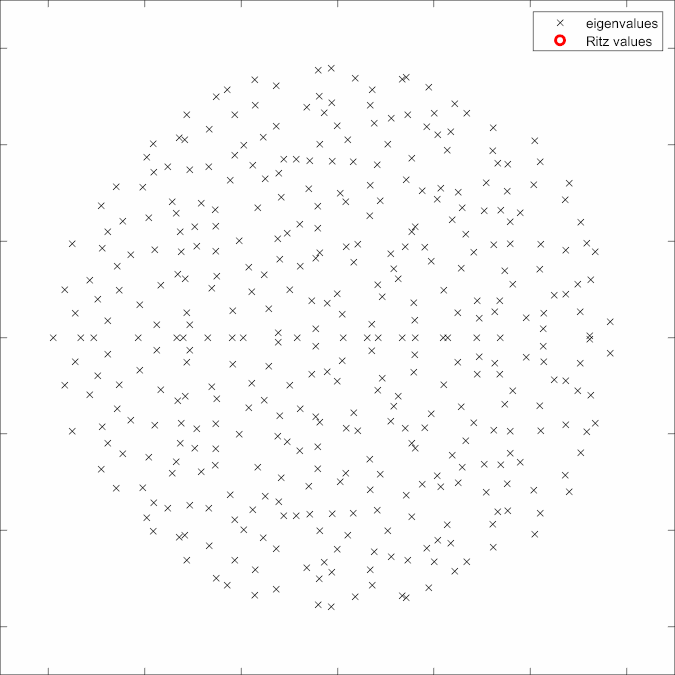top of page## Control of Quantum SystemsControlling a process at the atomic and molecular scale requires an ability to manipulate the electromagnetic field effectively. In the case of spins, whether they are used for building a quantum computer or in the design of a better NMR (or MRI), we can exert control by manipulating the magnetic field. In femtochemistry and atomic physics, one uses lasers to exert control on the wavefunction.

This animation shows a number of spins that start out pointing in the +Z direction, and under the effect of a specially designed pulse, move to the +Y direction.

This project is about designing optimization approaches and faster solvers that could be used for designing optimal control pulses.

Areas: optimal control, numerical optimization

## Approximating the Matrix ExponentialThe solution to a linear PDE

u' = A u

such as the Schrödinger equation can be expressed explicitly in terms of the exponential of A,

u(t) = exp(t A) u(0).

We may approximate the exponential by truncating the Taylor expansion. These are polynomial approximations. The first such truncation is the Forward Euler method, which suffers from stability issues. Krylov subspace methods are among the most flexible polynomial approximations and have superlinear convergence.

However, the Ritz values (which are the Krylov approximation of the eigenvalues) start accumulating at the eigenvalues from the outer part of the spectrum - the animation shows how Ritz values accumulate for a random matrix. This means, as the norm of the matrix grows, we either need a large number of Krylov iterations or very small time-steps.

I am interested in the design of rational approximations, exponential splittings and Krylov methods that can allow us to take much larger steps and lead to efficient approximation of the exponential.

Areas: rational approximation, Krylov methods, exponential splittings

## Geometric Numerical IntegrationSymmetries in a physical system lead to conservation laws such as conservation of mass, momentum, angular momentum and energy.

This animation shows the solution of the Schrödinger equation with radially symmetric initial conditions. The radial symmetry of the system results in conservation of angular momentum. Do the numerical methods we use for simulating this system conserve the angular momentum?

Geometric Numerical Integration is about the design and analysis of methods that can conserve some of these properties exactly or approximately.

Areas: Lie algebras/groups, exponential splittings, convergence analysis.

Disclaimer: These are somewhat outdated descriptions and don't cover some of the more active areas of my interest. If you are interested in doing a project with me, please reach out at ps2106@bath.ac.uk.

bottom of page